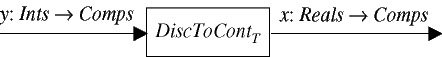EECS20N: Signals and Systems

# Reconstruction

Given a discrete-time signal y we can construct a continuous-time signal using a system

DiscToContT : DiscSignalsContSignals.

In pictures,This system can be realized in any number of ways. Some examples include:

• Zero-order hold. This means simply that the value of the each sample y(n) is held constant for duration T, so that x(t) = y(n) for the time interval from t = nT to t = (n + 1)T. Let this system be denoted

ZeroOrderHoldT : DiscSignalsContSignals.

• Linear interpolation. Intuitively, this means simply that we connect the dots with straight lines. Specifically, in the time interval from t = nT to t = (n + 1)T, x(t) has values that vary along a straight line from y(n) to y(n+1). Linear interpolation is sometimes called first-order hold. Let this sytem be denoted

LinearInterpolatorT : DiscSignalsContSignals.

• Ideal interpolation. It is not at all clear what this should mean, but intuitively, it should result in a smooth curve that passes through the samples. We will give a precise meaning below. Let this system be denoted

IdealInterpolatorT : DiscSignalsContSignals.

These methods are demonstrated by the following applet.

If you were able to run applets, you would have one here.

The selection box to the right can be used to choose the reconstruction method. The top plot is a time-domain plot that makes it clear what each method means. The discrete-time signal is shown in red and the continuous-time reconstructed signal in blue. The bottom plot gives a frequency domain representation of the reconstructed signal. Notice that if the reconstruction method is "ideal" then the frequency domain representation is exactly that of a sinusoid.

However, for each of the other methods, the reconstructed signal is not exactly a sinusoid. For linear interpolation, it is close, but if you look closely, you can see that there are some spurious terms in the frequency domain. Look, for example, in the vicinity of 8,500 Hz. For zero-order hold, these spurious terms are much bigger. For impulses, the spurious terms become as large as the ideal terms.

A smooth interpolation between samples, therefore, corresponds to minimizing the high frequency terms. In fact, it corresponds to eliminating all the frequency components above the Nyquist frequency. Thus, if we sample a signal and then reconstruct it using ideal interpolation, the result is the same as the original signal only if the original signal has no frequency components above the Nyquist frequency.